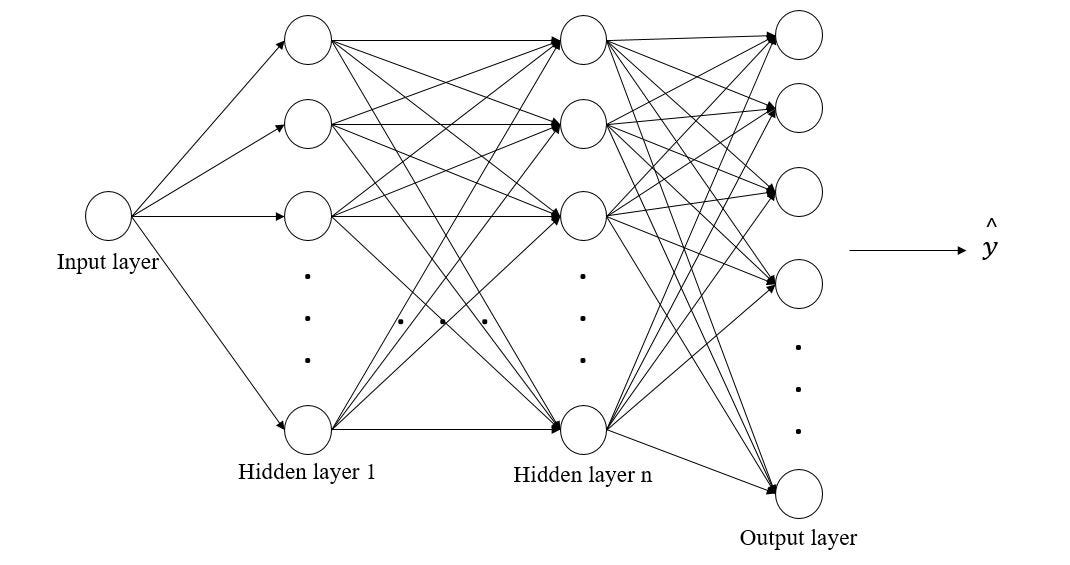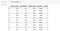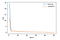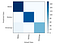# PyTorch vs Tensorflow (Deep Learning Model)

“Classical machine learning relies on using statistics to determine relationships between features and labels and can be very effective for creating predictive models. However, massive growth in the availability of data coupled with advances in the computing technology required to process it has led to the emergence of new machine learning techniques that mimic the way the brain processes information in a structure called an artificial neural network.”Deep Neural Network!

From the title, I believe you guys can figure it out that we are going to work on both PyTorch and Tensorflow to build a simple deep learning model based on a single dataset. This article is going to be a comparison between these two modules. Alright, let’s get to the point! We are going to use Penguins Dataset!

import pandas as pd

data = pd.read_csv(‘./penguins.csv’).dropna()

data[‘FlipperLength’] = data[‘FlipperLength’]/10
data[‘BodyMass’] = data[‘BodyMass’]/100

for i in range(1,3):
data = data.append(data)Dataset!

In the above code, we have used panda to load the dataset. While loading the dataset, we have dropped the rows that contain null values! As I have already seen the dataset before. I have also normalized the dataset. It’s always a good practice to normalize your dataset to put the features into the same space. It helps to build a better model. My selected dataset is pretty smaller so I have increased it manually. However, the code for these steps is the same for both PyTorch and Tensorflow!

`data.shape:(1368, 5)`

from sklearn.model_selection import train_test_split

features = [‘CulmenLength’, ‘CulmenDepth’, ‘FlipperLength’, ‘BodyMass’]
labels = ‘Species’

x_train, x_test, y_train, y_test = train_test_split(data[features].values, data[labels].values, test_size=0.30, random_state=0)

Next up, we have to split the dataset into training and testing sets. We have used 70% and 30% for training and testing set accordingly. The code for both modules is the same!

`print ('Training Set: %d, Test Set: %d \n' % (len(x_train), len(x_test)))Training Set: 957, Test Set: 411`

Installing PyTorch and Tensorflow:

1. !pip install torch==1.7.1+cpu torchvision==0.8.2+cpu torchaudio==0.7.2 -f https://download.pytorch.org/whl/torch_stable.html
2. !pip install — upgrade tensorflow

Loading the Libraries for both modules:

1. PyTorch:
`import torchimport torch.nn as nnimport torch.utils.data as td# Set random seed for reproducabilitytorch.manual_seed(0)print("Libraries imported - ready to use PyTorch", torch.__version__)Libraries imported - ready to use PyTorch 1.7.1+cpu`

2. Tensorflow:

`import tensorflowfrom tensorflow import kerasfrom tensorflow.keras import modelsfrom tensorflow.keras.models import Sequentialfrom tensorflow.keras.layers import Densefrom tensorflow.keras import utilsfrom tensorflow.keras import optimizers# Set random seed for reproducabilitytensorflow.random.set_seed(0)print("Libraries imported.")print('Keras version:',keras.__version__)print('TensorFlow version:',tensorflow.__version__)Libraries imported.Keras version: 2.2.4-tfTensorFlow version: 2.0.0-alpha0`

Now that we have everything we need, let’s build the model:

First up, we have to prepare the data for both modules!

PyTorch:

`# Preparing Train datatrain_x = torch.Tensor(x_train).float()train_y = torch.Tensor(y_train).long()train_ds = td.TensorDataset(train_x, train_y)train_loader = td.DataLoader(train_ds, batch_size=20, shuffle=False, num_workers=1)# Preparing Test datatest_x = torch.Tensor(x_test).float()test_y = torch.Tensor(y_test).long()test_ds = td.TensorDataset(test_x, test_y)test_loader = td.DataLoader(test_ds, batch_size=20, shuffle=False, num_workers=1)`

PyTorch makes use of data loaders to load training and validation data in batches. We need to wrap those in PyTorch datasets (in which the data is converted to PyTorch tensor objects) and create loaders to read batches from those datasets.

Tensorflow:

`# Set data types for float featuresx_train = x_train.astype('float32')x_test = x_test.astype('float32')# Set data types for categorical labelsy_train = utils.to_categorical(y_train)y_test = utils.to_categorical(y_test)`

In TensorFlow, we need to set the data type of our features to 32-bit floating-point numbers and specify that the labels represent categorical classes rather than numeric values.

Now, deep neural network model setup:

PyTorch:

`class NModel(nn.Module):    def __init__(self):        super(NModel, self).__init__()        self.fc1 = nn.Linear(len(features), 10)        self.fc2 = nn.Linear(10, 10)        self.fc3 = nn.Linear(10, 3)            def forward(self, x):        x = torch.relu(self.fc1(x))        x = torch.relu(self.fc2(x))        x = torch.softmax(self.fc3(x), dim=1)                return xmodel = NModel()print(model)Output:NModel(  (fc1): Linear(in_features=4, out_features=10, bias=True)  (fc2): Linear(in_features=10, out_features=10, bias=True)  (fc3): Linear(in_features=10, out_features=3, bias=True))`

The neural network concept is the same for both modules! The way of writing is different. In PyTorch, We need to create a class where we have to initialize our model layers and neurons in each layer. In this simple model, we created three layers, a neural network model. The First layer takes input based on the features space, and we set 10 neurons for both the first and second hidden layers. The third layer is the output layer which will produce the label spaces. In this case, the output space is 3! The first two layers will use the ReLU activation function and the output layer will use the Softmax activation function!

Tensorflow:

`model = Sequential()model.add(Dense(10, input_dim=len(features), activation='relu'))model.add(Dense(10, input_dim=10, activation='relu'))model.add(Dense(len(penguin_classes), input_dim=10, activation='softmax'))print(model.summary())Model: "sequential"_________________________________________________________________Layer (type)                 Output Shape              Param #   =================================================================dense (Dense)                (None, 10)                50        _________________________________________________________________dense_1 (Dense)              (None, 10)                110       _________________________________________________________________dense_2 (Dense)              (None, 3)                 33        =================================================================Total params: 193Trainable params: 193Non-trainable params: 0_________________________________________________________________None`

In Tensorflow, we have to use the sequential model in this case. In the sequential model, we used dense layers with 10 neurons! If you compare the both models for PyTorch and Tensorflow, then you will be able to figure out that both model structure is same but representation is different! So, which module is my favorite? Obviously, Tensorflow because of fewer codes! 😆

Alright, so far we have pre-processed the dataset to feed into the deep learning model based on PyTorch and Tensorflow. Let’s run and test them!

PyTorch:

1. Train:
`def train(model, data_loader, optimizer):    # Set the model to training mode    model.train()    train_loss = 0        for batch, tensor in enumerate(data_loader):        data, target = tensor        # feedforward step        optimizer.zero_grad()        out = model(data)        loss = loss_criteria(out, target)        train_loss += loss.item()        # backpropagation to improve the model performances        loss.backward()        optimizer.step()    # Return average loss of the training     avg_loss = train_loss / (batch+1)    print('Training set: Average loss: {:.6f}'.format(avg_loss))    return avg_loss`

2. Test:

`def test(model, data_loader):    model.eval()    test_loss = 0    correct = 0with torch.no_grad():        batch_count = 0        for batch, tensor in enumerate(data_loader):            batch_count += 1            data, target = tensor            # Get the predictions            out = model(data)            # calculate the loss            test_loss += loss_criteria(out, target).item()            # Calculate the accuracy            _, predicted = torch.max(out.data, 1)            correct += torch.sum(target==predicted).item()                # Calculate the average loss and total accuracy for this epoch    avg_loss = test_loss/batch_count    print('Validation set: Average loss: {:.6f}, Accuracy: {}/{} ({:.0f}%)\n'.format(        avg_loss, correct, len(data_loader.dataset),        100. * correct / len(data_loader.dataset)))        # return average loss for the epoch    return avg_loss`

3. Additional Setup:

`# Specify the loss criteria (CrossEntropyLoss for multi-class classification)loss_criteria = nn.CrossEntropyLoss()# We are using Adam optimizerlearning_rate = 0.001 # try different learning rateoptimizer = torch.optim.Adam(model.parameters(), lr=learning_rate) # setting the Adam algorithmoptimizer.zero_grad()# We'll be saving metrics for each epoch in these arraysepoch_nums = []training_loss = []validation_loss = []# Train over 50 epochsepochs = 50for epoch in range(1, epochs + 1):    print('Epoch: {}'.format(epoch))        # Feed training data into the model to optimize the weights    train_loss = train(model, train_loader, optimizer)        # Feed the test data into the model to check its performance    test_loss = test(model, test_loader)        # Log the metrics for this epoch    epoch_nums.append(epoch)    training_loss.append(train_loss)    validation_loss.append(test_loss)`

4. Model outputs:

`Epoch: 47Training set: Average loss: 0.569989Validation set: Average loss: 0.572491, Accuracy: 408/411 (99%)Epoch: 48Training set: Average loss: 0.569646Validation set: Average loss: 0.572059, Accuracy: 408/411 (99%)Epoch: 49Training set: Average loss: 0.569319Validation set: Average loss: 0.571643, Accuracy: 408/411 (99%)Epoch: 50Training set: Average loss: 0.569007Validation set: Average loss: 0.571244, Accuracy: 408/411 (99%)`

Tensorflow:

1. Train and Test:
`learning_rate = 0.001 # learning rateopt = optimizers.Adam(lr=learning_rate) # model optimizer# compiling the model with CC loss and optimizer! Along with Acc. metrics!model.compile(loss='categorical_crossentropy',              optimizer=opt,              metrics=['accuracy'])# we will do 50 sets of run and in epochs, 10 will be the batch sizenum_epochs = 50history = model.fit(x_train, y_train, epochs=num_epochs, batch_size=10, validation_data=(x_test, y_test))`

2. Model Outputs:

`Epoch 47/50957/957 [==============================] - ETA: 0s - loss: 0.1854 - accuracy: 0.90 - ETA: 0s - loss: 0.1081 - accuracy: 0.97 - 0s 109us/sample - loss: 0.1073 - accuracy: 0.9739 - val_loss: 0.1230 - val_accuracy: 0.9830Epoch 48/50957/957 [==============================] - ETA: 0s - loss: 0.0697 - accuracy: 1.00 - ETA: 0s - loss: 0.1037 - accuracy: 0.97 - 0s 116us/sample - loss: 0.0994 - accuracy: 0.9812 - val_loss: 0.1116 - val_accuracy: 0.9805Epoch 49/50957/957 [==============================] - ETA: 0s - loss: 0.0896 - accuracy: 1.00 - ETA: 0s - loss: 0.1031 - accuracy: 0.97 - 0s 122us/sample - loss: 0.0968 - accuracy: 0.9770 - val_loss: 0.1008 - val_accuracy: 0.9830Epoch 50/50957/957 [==============================] - ETA: 0s - loss: 0.0447 - accuracy: 1.00 - ETA: 0s - loss: 0.0942 - accuracy: 0.97 - 0s 126us/sample - loss: 0.0922 - accuracy: 0.9833 - val_loss: 0.1066 - val_accuracy: 0.9781`

So far, we have seen the difference between PyTorch and Tensorflow to run the model. Let’s discuss it: “In each epoch, the full set of training data is passed forward through the network. In each epoch, 10 batches of data are provided. Typically, a neural network consists of forwarding propagation and backpropagation. In each layer, each neuron uses an activation function to produce neurons' weights and biases! At the output layer, the loss function is used to validate model performances. That’s where the backpropagation comes in. Backpropagation is useful to improve model weights and biases, which eventually helps to produce a better model. Also, At the end of each epoch, the validation data is passed through the network, and its loss and accuracy are also calculated. It’s important to do this because it enables us to compare the performance of the model using data on which it was not trained, helping us determine if it will generalize well for new data or if it’s overfitted to the training data.”

Visualize the training and validation Phase:

`%matplotlib inlinefrom matplotlib import pyplot as pltepoch_nums = range(1,num_epochs+1)training_loss = history.history["loss"]validation_loss = history.history["val_loss"]plt.plot(epoch_nums, training_loss)plt.plot(epoch_nums, validation_loss)plt.xlabel('epoch')plt.ylabel('loss')plt.legend(['training', 'validation'], loc='upper right')plt.show()`

The output:Model Training and Validations

PyTorch:

for param_tensor in model.state_dict():
print(param_tensor, “\n”, model.state_dict()[param_tensor].numpy())

`fc1.weight  [[-0.00374341  0.2682218  -0.41152257 -0.3679695 ] [-0.20487966 -0.28398493  0.13597898  0.6053104 ] [-0.04437202  0.13230628 -0.15110654 -0.09828269] [-0.47767425 -0.33114105 -0.20611155  0.01852179] [ 0.17907079  0.28123733 -0.35748705 -0.23587279] [ 0.40088734  0.382148   -0.20181231  0.3537034 ] [-0.08059168  0.05290705  0.4527381  -0.46383518] [-0.35555413 -0.16605727 -0.23530953  0.38971528] [-0.32408983 -0.23016644 -0.34932023 -0.4682805 ] [-0.43653095  0.80248505  0.29239044  0.17577983]]fc1.bias  [ 0.02629578 -0.26949045  0.08459234 -0.46684736 -0.3798124  -0.4262236  0.31546897  0.25337356 -0.22174752  0.2345465 ]fc2.weight  [[ 2.02246875e-01  3.14372510e-01  1.25505149e-01  4.27201092e-02   2.12026387e-01 -1.86195642e-01  5.89271486e-02 -2.45173126e-01  -2.19173074e-01 -1.63358063e-01] [ 1.43084526e-01  1.60798699e-01 -1.87318310e-01  9.55346525e-02   1.92188621e-01  1.52368024e-01  1.20740533e-02  4.16618437e-02   1.96180314e-01  9.25334394e-01] [-2.43692577e-01 -1.43560484e-01  1.24280632e-01  2.62010306e-01   2.59306699e-01  3.23746145e-01  6.29339218e-02 -2.45525643e-01   2.90905833e-02 -6.68823123e-01] [-2.94709772e-01  5.11151612e-01  2.40446895e-01 -3.15446049e-01   5.91889918e-02 -1.04206558e-02 -5.20388186e-02 -1.01968892e-01   1.21607333e-01 -4.82648820e-01] [ 1.15926355e-01  1.59918934e-01  2.26378471e-01  1.18241072e-01  -3.12981755e-01 -2.05135971e-01  1.57897264e-01  6.61869049e-02  -2.46684223e-01 -1.82090104e-01] [ 2.97491044e-01  5.08512676e-01 -1.37883261e-01 -7.95897096e-02  -3.19968253e-01 -9.41922441e-02 -2.38138139e-01 -2.13026911e-01  -1.74240172e-02 -3.14111978e-01] [-1.29504845e-01  1.87642485e-01 -1.92436963e-01  2.86935598e-01   2.16710836e-01 -2.66669482e-01 -7.87041336e-02  1.42690241e-02   4.61379588e-02  7.50010908e-02] [ 1.24096721e-01  1.89420879e-02 -1.54296622e-01  1.49635494e-01  -3.03341120e-01 -1.87430307e-01 -7.91612566e-02 -1.54038772e-01  -1.10627025e-01 -2.59187132e-01] [-6.72664344e-02  3.37419212e-01 -2.06011564e-01 -1.62286162e-02   2.08991051e-01 -1.28818750e-01  8.78867507e-03  8.23016744e-04   6.39986098e-02  2.39946589e-01] [ 2.99545556e-01  2.00822324e-01  3.00230891e-01 -2.28701234e-02  -2.84074187e-01 -1.49916381e-01  2.15321153e-01 -2.04995275e-03  -1.57179862e-01 -2.42329061e-01]]fc2.bias  [-0.2959424   0.13159421 -0.27384382  0.07626942  0.17096573 -0.4653062  0.19725719 -0.24745122 -0.09499434 -0.1282217 ]fc3.weight  [[-0.06091028  0.32780746 -0.9960096  -1.0807507  -0.04948315 -0.3577183  -0.14365433  0.11912274  0.19421846 -0.02134135] [ 0.27809682 -0.47728527  0.09838016  0.83235973 -0.2853832   0.9088981  -0.03649095 -0.14116624  0.38886335 -0.25554216] [ 0.03393281 -0.19362502  1.0301037  -0.24135342  0.15194914 -0.6721673  -0.07604478 -0.06650442 -1.1390747   0.17134616]]fc3.bias  [ 0.5242965  -0.09666859 -0.13340557]`

Tensorflow:

for layer in model.layers:
weights = layer.get_weights()
biases = layer.get_weights()
print(‘ — — — — — — \nWeights:\n’,weights,’\nBiases:\n’, biases)

`------------Weights: [[-0.27236846 -0.3841947   0.03234848  0.08020484 -0.10909867  0.06330433  -0.19313203  0.84640336  0.36701852 -0.4905011 ] [ 0.27471453  0.21265197  0.08079417 -0.17707926 -0.10406601  0.80510986   0.3481228  -0.04539559 -0.62267196 -0.5447268 ] [-0.28836262 -0.634329    0.28234363  0.34767175  0.23550075 -0.00817027   0.14570452 -0.7969777  -0.5233428   0.3296095 ] [-0.42851955 -0.24623463 -0.2871196  -0.5230521  -0.43773973  0.37682083  -0.0763066   0.23378253  0.74960405 -0.4691702 ]] Biases: [ 0.          0.         -0.01398832  0.          0.          0.20292243 -0.19176428 -0.2576086  -0.3294029   0.        ]------------Weights: [[ 0.0607031  -0.30530828  0.39975524  0.3037489   0.15896738  0.03326017  -0.53190327  0.40915883 -0.03316814 -0.1240823 ] [ 0.42301047  0.14984506 -0.54566675  0.3919103  -0.4295466   0.50397205  -0.31616646  0.17803025 -0.41518384 -0.38429344] [ 0.5336163   0.37752342 -0.46671256  0.17206895 -0.04215616  0.5297911   0.4396385   0.2792814   0.2627702  -0.2233491 ] [-0.04491103  0.19579428 -0.26655364  0.17358297  0.3112036   0.53520477  -0.3109483  -0.5284722  -0.00098199 -0.44063687] [ 0.5135      0.39074183  0.39206952 -0.03048635  0.02663547  0.20555359   0.09307003  0.24590033 -0.49007446 -0.2917699 ] [ 0.50351286 -0.3901862   0.6034433   0.29417115 -0.26569188 -0.5313456   0.41638133 -0.15550071  0.00485109 -0.04526619] [ 0.26545775 -0.19090915  0.06236434 -0.30627972  0.12806404 -0.38203925  -0.21348043  0.41777134  0.26362625 -0.51192576] [-0.43856043 -0.21495155 -0.1461127  -0.46094683 -0.20800981  0.3025053  -0.00639509  0.40292972  0.2737926  -0.2580092 ] [-0.5078605  -0.4176368  -0.24811995 -0.49696004 -0.27225545 -0.38996994  -0.4037082   0.16782355 -0.2606465   0.22881041] [ 0.32320213 -0.30822456 -0.37115166  0.45703936 -0.35191107  0.24120325  -0.2000556   0.23292273 -0.33508268 -0.51532805]] Biases: [-0.03098752  0.          0.44007653  0.          0.          0.  0.13158555 -0.28722948 -0.30139711 -0.01467387]------------Weights: [[-0.38429576  0.3262583  -0.04640121] [ 0.50995994 -0.12620813 -0.6595991 ] [ 0.54413134  0.01471629 -0.22374861] [ 0.54463303 -0.50025463  0.06109887] [ 0.26757038 -0.67376095 -0.18467396] [ 0.08888024 -0.2536324   0.20257705] [ 1.1826342  -1.5543535   0.6450739 ] [-0.48484278  0.38147986  0.19515398] [-0.51660526 -0.35239688  0.6803643 ] [ 0.6415732  -0.61359274 -0.03051702]] Biases: [ 0.3130718   0.48137134 -0.49553102]`

PyTorch and Tensorflow:

`import numpy as npfrom sklearn.metrics import confusion_matriximport matplotlib.pyplot as plt%matplotlib inlineclass_probabilities = model.predict(x_test)predictions = np.argmax(class_probabilities, axis=1)true_labels = np.argmax(y_test, axis=1)# Plot the confusion matrixcm = confusion_matrix(true_labels, predictions)plt.imshow(cm, interpolation="nearest", cmap=plt.cm.Blues)plt.colorbar()tick_marks = np.arange(len(penguin_classes))plt.xticks(tick_marks, penguin_classes, rotation=85)plt.yticks(tick_marks, penguin_classes)plt.xlabel("Actual Class")plt.ylabel("Predicted Class")plt.show()`Confusion Matrix!

The confusion matrix should show a strong diagonal line indicating that there are more correct than incorrect predictions for each class. From the confusion matrix, it is also identified that the model is perfect in this example and produced almost 99% model accuracy.

That’s it for this article. I hope the comparison between PyTorch and Tensorflow is useful! I will be back with a convolutional neural network in the next article. Cheers! 😃

Data Scientists / Full Stack Deep Learning

Data Scientists / Full Stack Deep Learning